# 1. Determine the constant c such that the given function is a valid joint PDF for...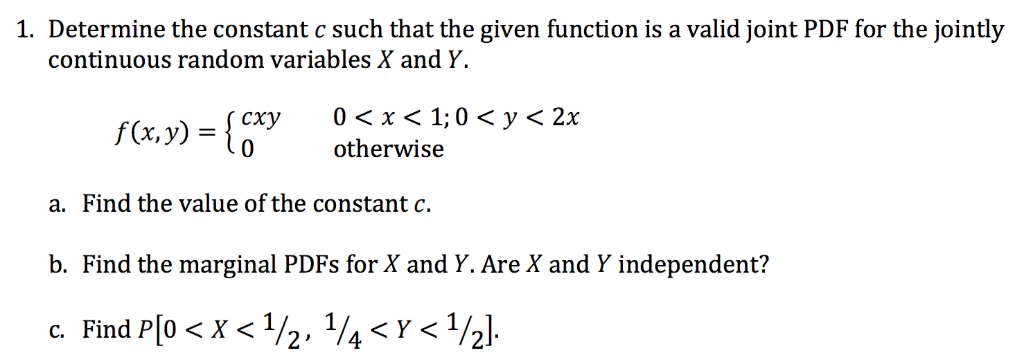1. Determine the constant c such that the given function is a valid joint PDF for the jointly continuous random variables X and Y. f(x,y) ={cry otherwise 0 < y < 2x a. Find the value of the constant c. b. Find the marginal PDFs for X and Y. Are X and Y independent?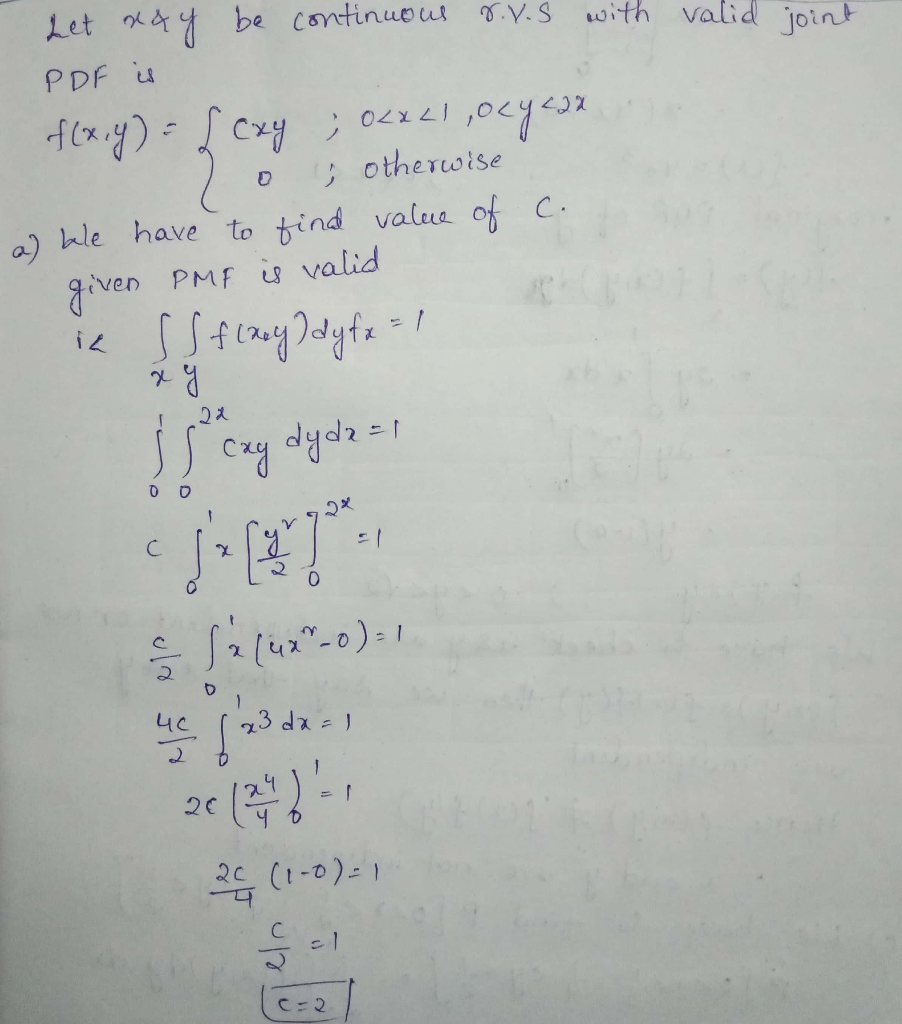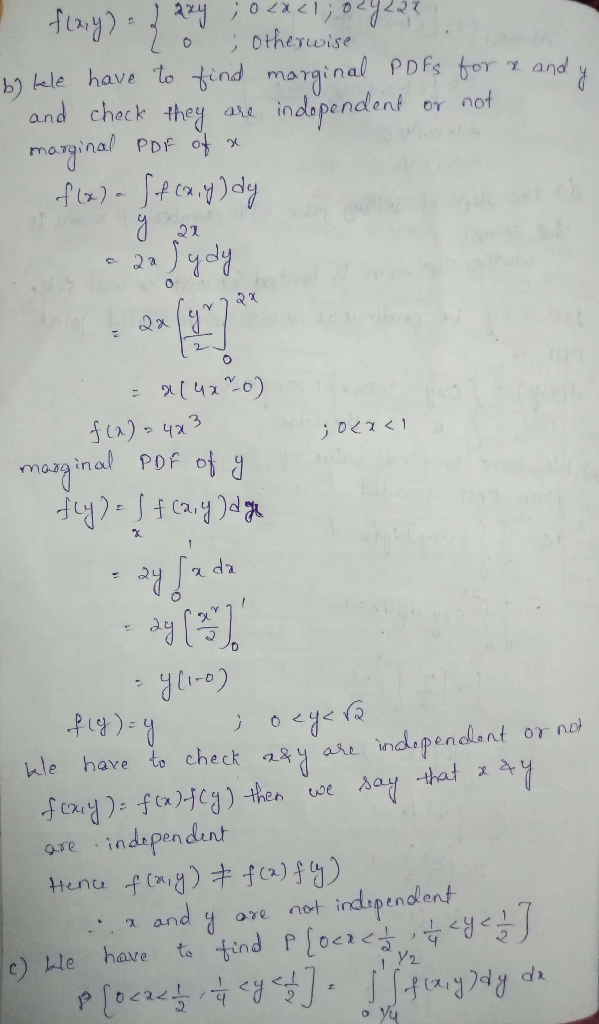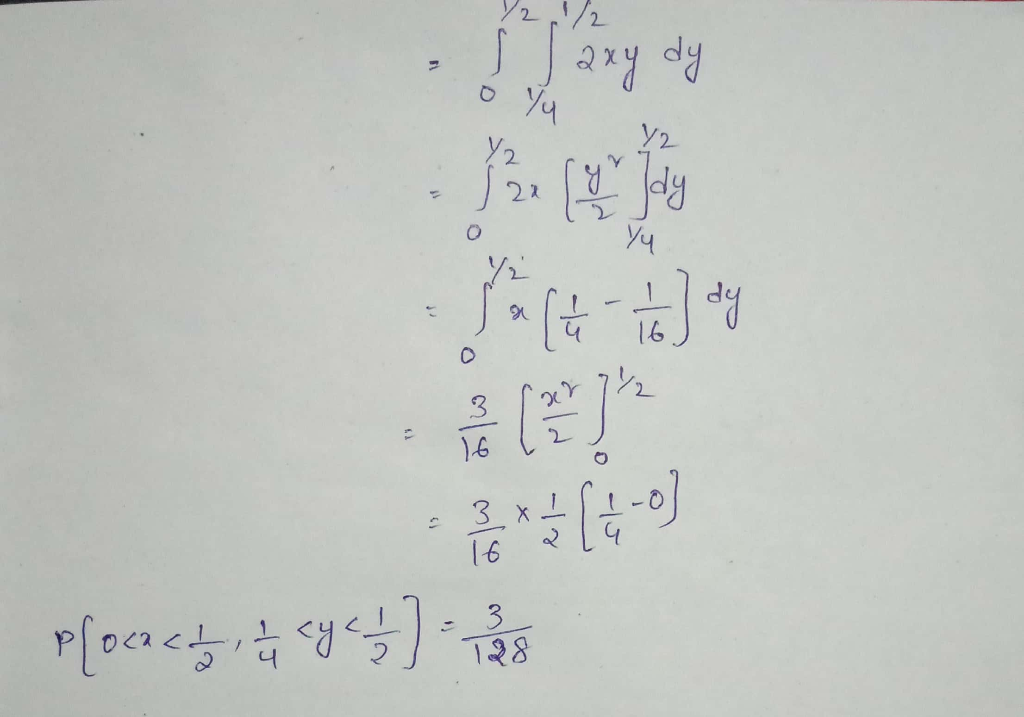#### Earn Coin

Coins can be redeemed for fabulous gifts.

Similar Homework Help Questions
• ### 2. Let the joint pdf of X and Y be given by f(xy)-cx if 0sysxsi Determine that value of c that makes f into a valid pdf. a. Find Pr(r ) b 2 C. Find Prl X d. Find the marginal pdf's of X and Y e....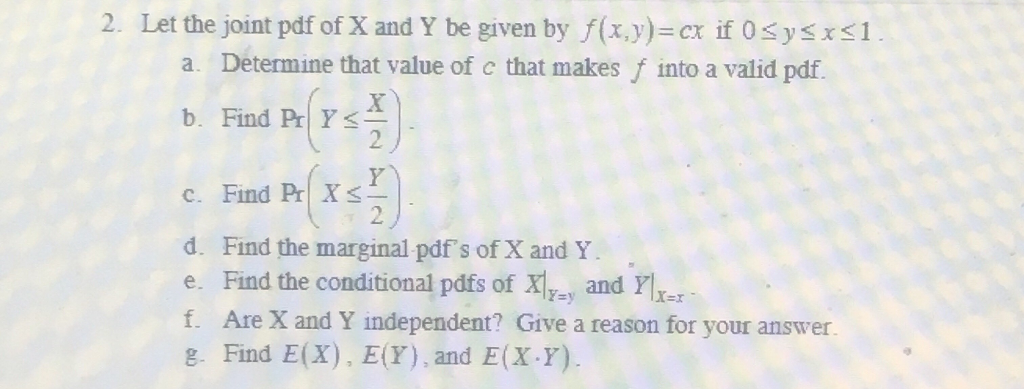2. Let the joint pdf of X and Y be given by f(xy)-cx if 0sysxsi Determine that value of c that makes f into a valid pdf. a. Find Pr(r ) b 2 C. Find Prl X d. Find the marginal pdf's of X and Y e. Find the conditional pdfs of 자리 and ri- f. Are X and Y independent? Give a reason for your answer g. Find E(X), E(Y), and E(X.Y) 2. Let the joint pdf of X...

• ### Q2) (20 points) The joint pdf of a two continuous random variables is given as follows:...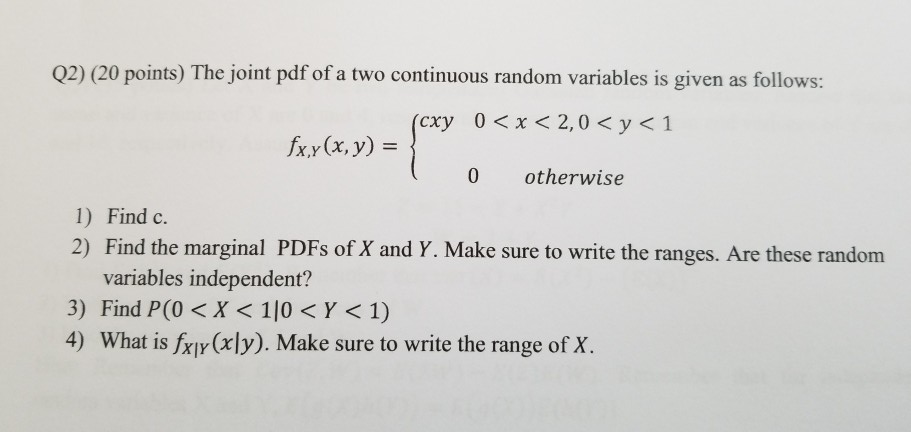Q2) (20 points) The joint pdf of a two continuous random variables is given as follows: < x < 2,0 < y<1 (cxy0 fxy(x, y) = } ( 0 otherwise 1) Find c. 2) Find the marginal PDFs of X and Y. Make sure to write the ranges. Are these random variables independent? 3) Find P(0 < X < 110 <Y < 1) 4) What is fxy(x\y). Make sure to write the range of X.

• ### 2. The joint pdf of random variables X and Y is given by f(x.y) k if...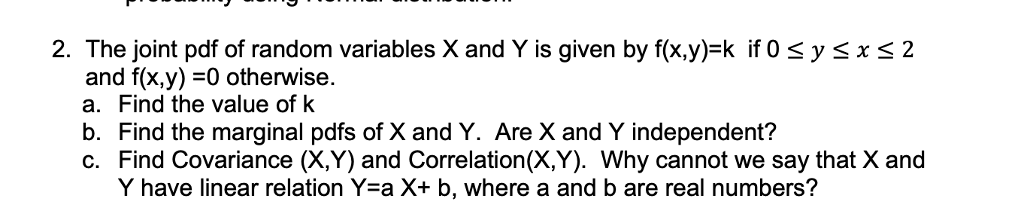2. The joint pdf of random variables X and Y is given by f(x.y) k if 0 sysxs2 and f(x,y)-0 otherwise. a. Find the value of k b. Find the marginal pdfs of X and Y. Are X and Y independent? c. Find Covariance (X,Y) and Correlation(X,Y). Why cannot we say that X and Y have linear relation Yea X+ b, where a and b are real numbers?

• ### Let the random variables X, Y with joint probability density function (pdf) fxy(z, y) = cry,...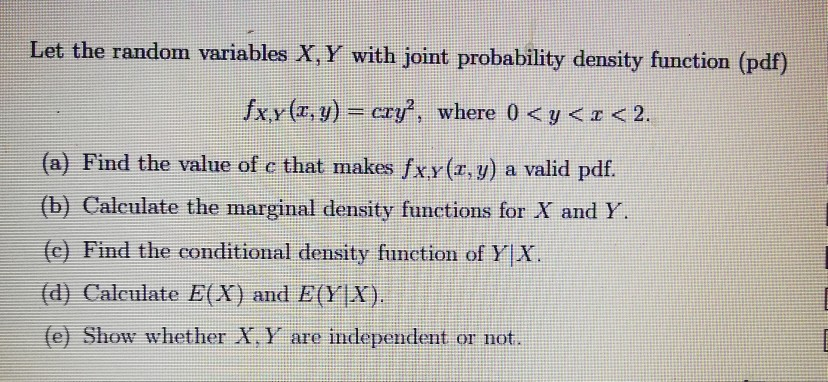Let the random variables X, Y with joint probability density function (pdf) fxy(z, y) = cry, where 0 < y < z < 2. (a) Find the value of c that makes fx.y (a, y) a valid pdf. (b) Calculate the marginal density functions for X and Y (c) Find the conditional density function of Y X (d) Calculate E(X) and EYIX) (e Show whether X. Y are independent or not.

• ### The joint pdf of random variables X and Y is given by f(x.y)-k if 0 s...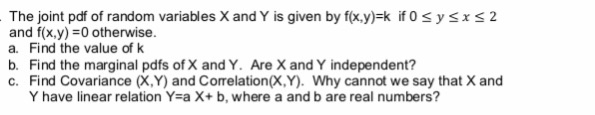The joint pdf of random variables X and Y is given by f(x.y)-k if 0 s y sx s 2 and f(x,y) =0 otherwise. a. Find the value of k b. Find the marginal pdfs of X and Y. Are X and Y independent? c. Find Covariance (X,Y) and Correlation(X,Y). Why cannot we say that X and Y have linear relation Y-a X+ b, where a and b are real numbers?

• ### Suppose the joint pdf of random variables X and Y is f(x,y) = c/x, 0 <...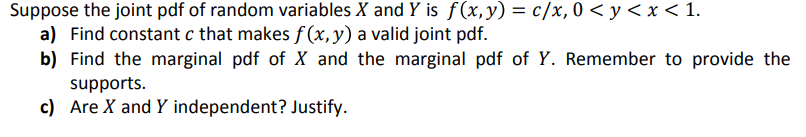Suppose the joint pdf of random variables X and Y is f(x,y) = c/x, 0 < y < x < 1. a) Find constant c that makes f (x, y) a valid joint pdf. b) Find the marginal pdf of X and the marginal pdf of Y. Remember to provide the supports c) Are X and Y independent? Justify

• ### 1. The joint probability density function (pdf) of X and Y is given by fxy(x, y)...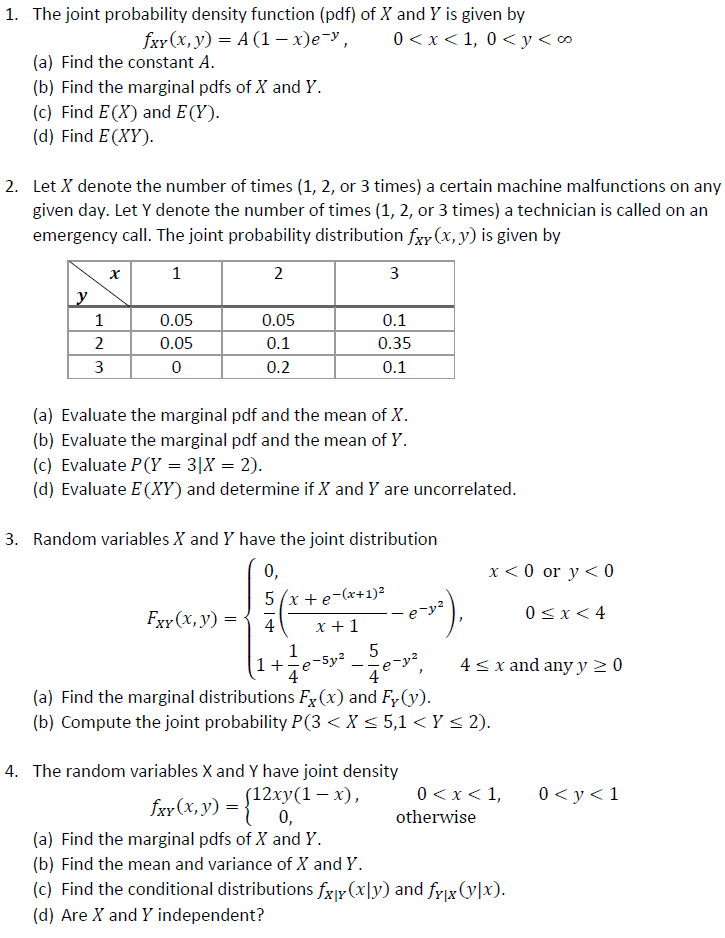1. The joint probability density function (pdf) of X and Y is given by fxy(x, y) = A (1 – xey, 0<x<1,0 < y < 0 (a) Find the constant A. (b) Find the marginal pdfs of X and Y. (c) Find E(X) and E(Y). (d) Find E(XY). 2. Let X denote the number of times (1, 2, or 3 times) a certain machine malfunctions on any given day. Let Y denote the number of times (1, 2, or 3...

• ### The joint pdf fr (x)) of two random variables X and Y is given by fo...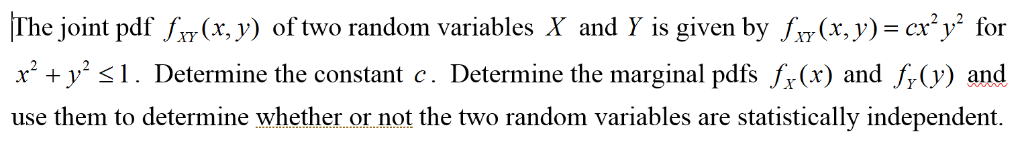The joint pdf fr (x)) of two random variables X and Y is given by fo (x,y)=cx2y for x +y s1. Determi use them to determine whether or not the two random variables are statistically independent. ne the constant c. Determine the marginal pdfs "Ax) and f, (y) and

• ### The joint PDF of random variables X and Y is expressed as (a) Determine the constant...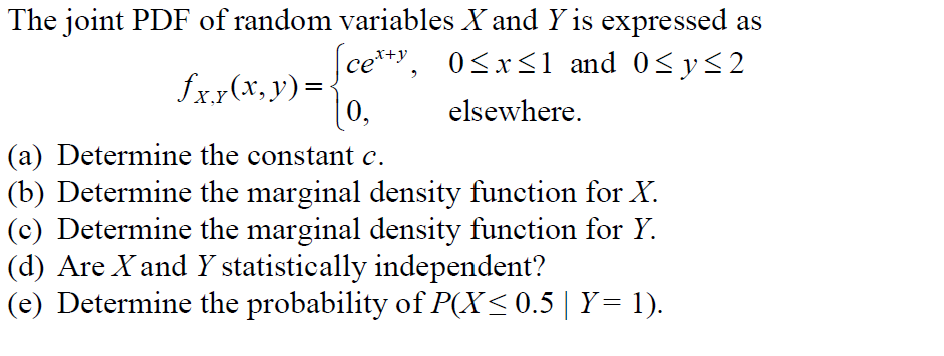The joint PDF of random variables X and Y is expressed as (a) Determine the constant c. (b) Determine the marginal density function for X. (c) Determine the marginal density function for Y. (d) Are X and Y statistically independent? (e) Determine the probability of P(X ≤ 0.5 | Y = 1). The joint PDF of random variables X and Y is expressed as certy, 05xs1 and 05ys2 fx.x(x, y) = 10. elsewhere. (a) Determine the constant c. (b) Determine...

• ### Please show your work. Thanks in advance. 3. The joint pdf for random variables X and...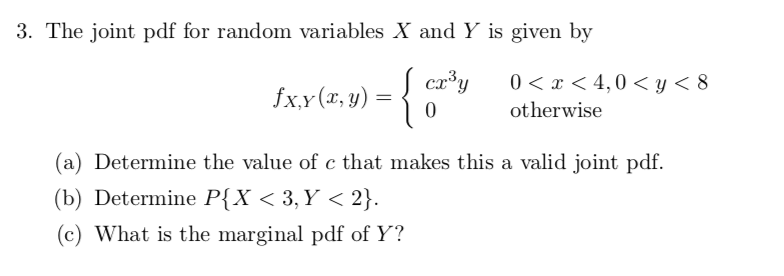Please show your work. Thanks in advance. 3. The joint pdf for random variables X and Y is given by 0 otherwise (a) Determine the value of c that makes this a valid joint pdf. (b) Determine P(X<3,Y< 2). (c) What is the marginal pdf of Y?